Quantified Self Experiments / QS - Nightly vs Standing HRV: sensivity to sickness

## Abstract

#### What did i do?

I've measured my HRV to find out if it is a useful indicator of sickness and have value in early sickness detection and indication of recovery.

#### How did i do it?

I've assessed morning sickness score, nightly HRV by using Oura ring and morning standing HRV by using Polar H10. Daily and 7-day rolling averages was used.

#### What did i learn?

Sickness substantially decreases my standing HRV and showing moderate decrease in my nightly HRV. Daily morning standing HRV seems to be more sensitive than nightly values and provides valuable feedback of sickness status. Standing RMSSD seems to be a useful tool which provides insights about illness onset and recovery and helps make a decision of resuming physical activity. Nightly RMSSD values also provides insights on sickness status, but less sensitive.

## Introduction

There is a some evidence that sickness cause drop in HRV.

The purpose of this observational data analysis (n=1) is to find if sickness causing decrease HRV.

## Materials & Methods

#### Participants

Adult male (n=1) anthropometrics was described in previous article

#### Experimental design

From 2020-09-19 to 2021-09-01 (284 days) subjective sickness score was assessed by using 1-item 7-point Likert scale (0-6) every morning and evening: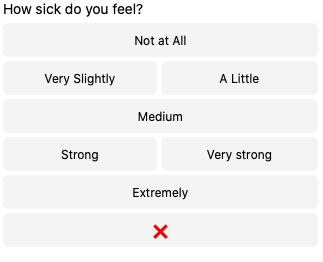Nightly HRV (RMSSD) was measured by Oura ring on a daily basis. 7-day rolling averages was calculated. Daily and 7-day values were log-transformed.

Morning HRV (RMSSD) was measured by Polar H10 in Kubios HRV app, after emptying urinary bladder, within 5 minutes after waking up. Stabilization period was 1 minute and measurement duration was 1 minute. 7-day rolling averages was calculated. Daily and 7-day values were log-transformed.

## Results

#### Sickness and HRV

Visual inspection of sickness status, daily RMSSD and 7D rolling averages.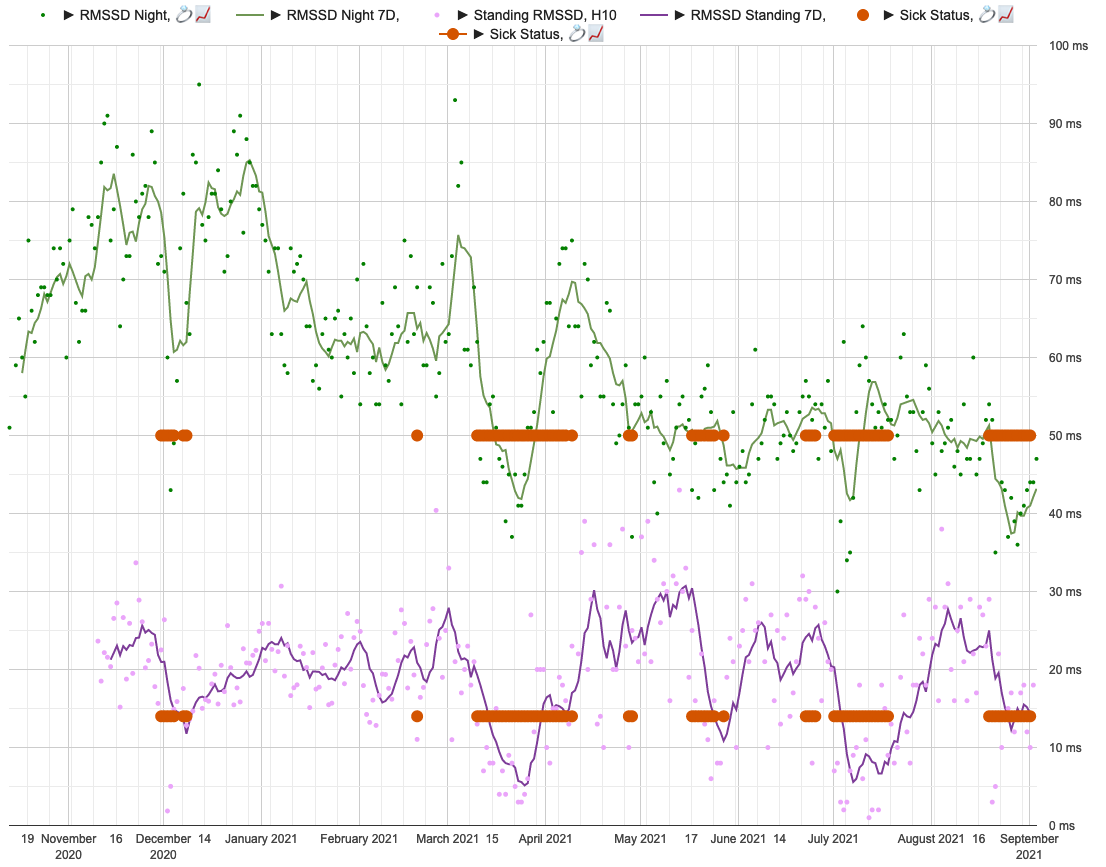Sickness status (red circles) for days with sickness score >= 6. Lines representing 7d rolling averages and points for daily values. Green for nightly and violet for standing values.

As we can see, HRV seems to drop significantly during sickness.

The data summary shown below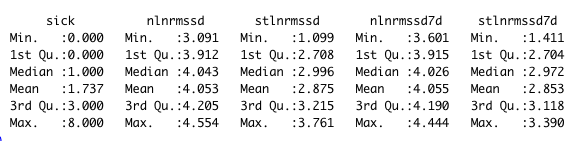sick is a sum of morning and evening scores. nlnmrssd - nightly ln(RMSSD). nlnmrssd7d - 7-day rolling average of nightly ln(RMSSD), stlnmrssd - morning standing ln(RMSSD). stlnmrssd7d - 7-day rolling average of morning standing ln(RMSSD).

Linear regression, Sick score

 effect p-adjusted effect size nightly ln(RMSSD) -0.051 <0.0001* moderate nightly 7d ln(RMSSD) -0.046 <0.0001* moderate standing ln(RMSSD) -0.145 <0.0001* substantial standing 7d ln(RMSSD) -0.118 <0.0001* substantial

We can see a moderate effect slopes for nightly values and substantial effect on a standing values. Morning standing RMSSD seems to be most sensitive to sickness status. Since my average standing ln(RMSSD) is 2.88 which is equivalent of RMSSD 17.8 ms, each 1 sickness point desceasing it by ~2.5 ms.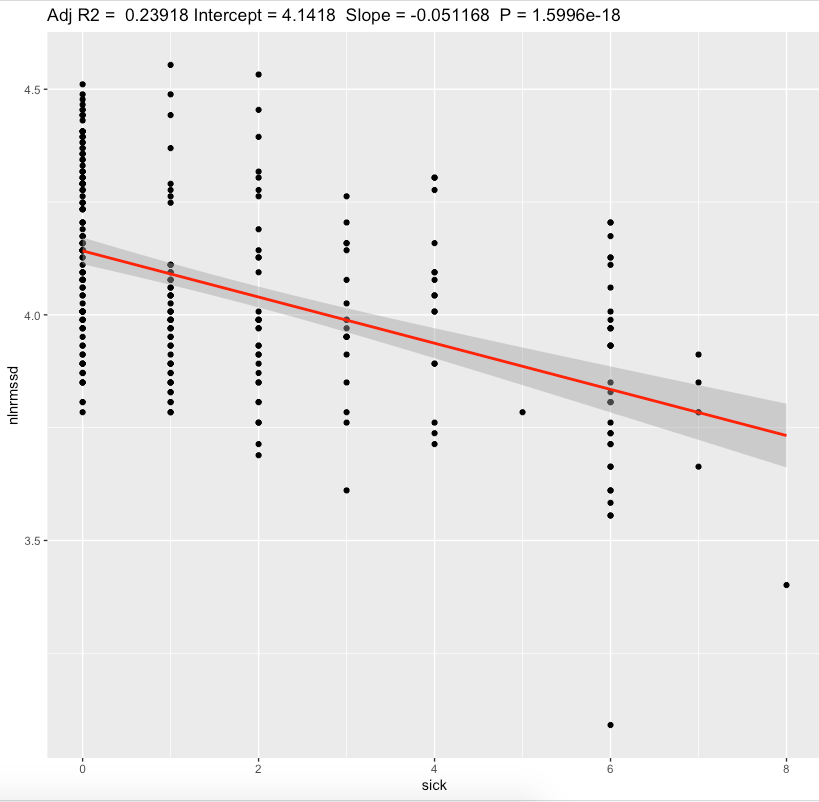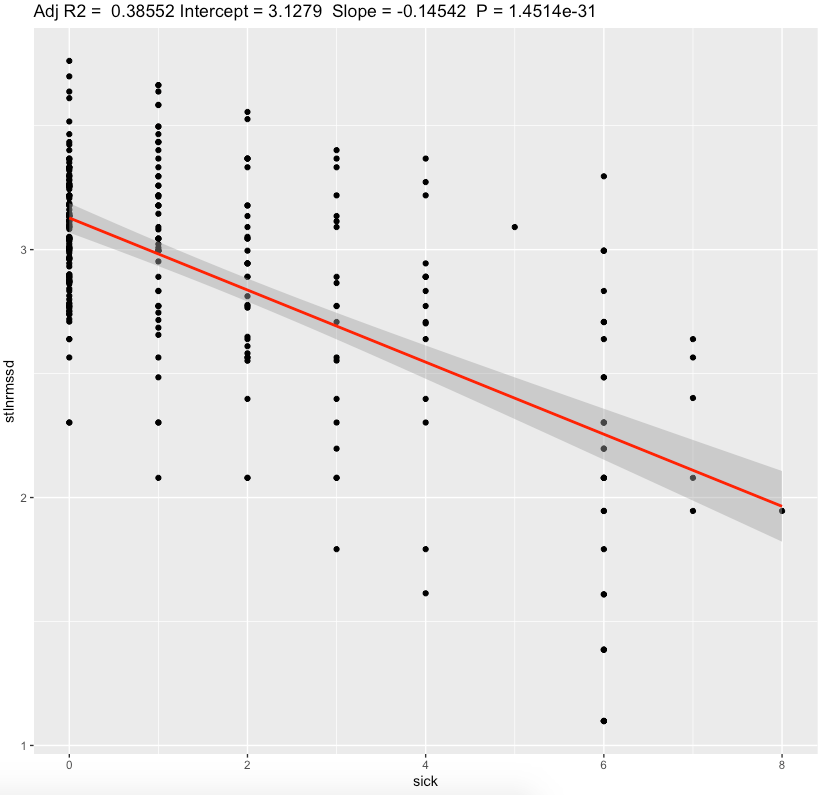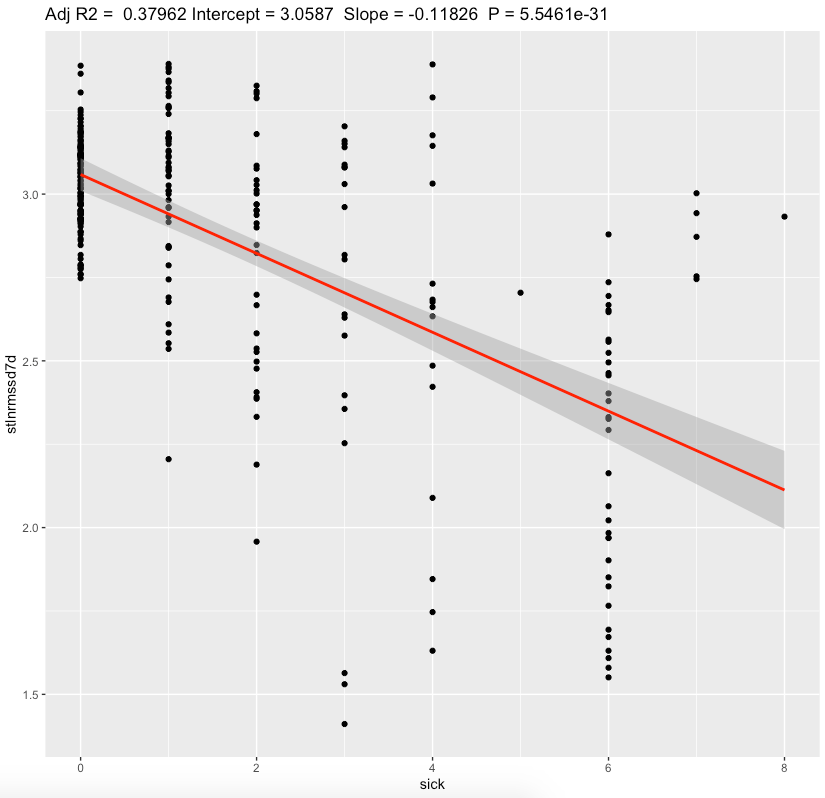## Discussion

The main result of this experiment is a statistically significant association between HRV and sickness with moderate/substantial effect sizes.

For 4-6 sickness score representing illness, standing RMSSD drop by ~10-15ms seems to be a huge. Nightly values are less sensitive.

In conclusion, these results points me to continue to measure my morning standing HRV and use it as possible indication of sickness onset and recovery status after illness. In my case, i prefer a standing HRV because of bigger sensitivy to sickness compared with nightly values.

Limitations:

• These results are NOT generalizable
• Absence of blinding, randomization, observational nature, n=1
• Often correlation ≠ causation
• Questionnaires widely used in research are prone to participant bias.
• There is a lot of other factors affecting HRV (sleep, diet, caffeine etc) which not taken into account

## Data availability & Information

Welcome for questions, suggestions and critics in comments below.

Data is fully available here

`library(dplyr)library(lubridate)library(effectsize)library(jsonlite)ggplotRegression <- function (fit) {    require(ggplot2)    ggplot(fit\$model, aes_string(x = names(fit\$model), y = names(fit\$model))) +    geom_point() +    stat_smooth(method = "lm", col = "red") +    labs(title = paste("Adj R2 = ",signif(summary(fit)\$adj.r.squared, 5),                       "Intercept =",signif(fit\$coef[],5 ),                       " Slope =",signif(fit\$coef[], 5),                       " P =",signif(summary(fit)\$coef[2,4], 5)))}daily <- read.csv("https://blog.kto.to/uploads/pa-na-rpe-sick-food-step-sauna-meditation-hrv.csv")daily <- daily[!is.na(daily\$`sick`),]daily <- daily[!is.na(daily\$`rpe`),]daily <- daily[!is.na(daily\$`rpe7d`),]daily <- daily[!is.na(daily\$`nlnrmssd`),]daily <- daily[!is.na(daily\$`nlnrmssd7d`),]daily <- daily[!is.na(daily\$`stlnrmssd`),]daily <- daily[!is.na(daily\$`stlnrmssd7d`),]daily\$pa = NULLdaily\$na = NULLdaily\$food = NULLdaily\$steps = NULLdaily\$sauna = NULLdaily\$meditation = NULLdaily\$rpe = NULLdaily\$rpe7d = NULLsummary(daily)l <- lm(cbind(nlnrmssd, nlnrmssd7d, stlnrmssd, stlnrmssd7d) ~ sick, data=daily)summary(daily)summary(anova(l))s <- summary(l); sinterpret_r2(s\$`Response nlnrmssd`\$adj.r.squared)interpret_r2(s\$`Response nlnrmssd7d`\$adj.r.squared)interpret_r2(s\$`Response stlnrmssd`\$adj.r.squared)interpret_r2(s\$`Response stlnrmssd7d`\$adj.r.squared)p.adjust(c(  s\$`Response nlnrmssd`\$coefficients[,4],  s\$`Response nlnrmssd7d`\$coefficients[,4],  s\$`Response stlnrmssd`\$coefficients[,4],  s\$`Response stlnrmssd7d`\$coefficients[,4]  ), method="BH")confint(l , level = 0.05)ggplotRegression(lm(nlnrmssd ~ sick, data=daily))ggplotRegression(lm(nlnrmssd7d ~ sick, data=daily))ggplotRegression(lm(stlnrmssd ~ sick, data=daily))ggplotRegression(lm(stlnrmssd7d ~ sick, data=daily))c <- cor.test(daily\$nlnrmssd, daily\$sick); cinterpret_r(c\$estimate[], "cohen1988")c <- cor.test(daily\$nlnrmssd7d, daily\$sick); cinterpret_r(c\$estimate[], "cohen1988")c <- cor.test(daily\$stlnrmssd, daily\$sick); cinterpret_r(c\$estimate[], "cohen1988")c <- cor.test(daily\$stlnrmssd7d, daily\$sick); cinterpret_r(c\$estimate[], "cohen1988")Response nlnrmssd :Call:lm(formula = nlnrmssd ~ sick, data = daily)Residuals:     Min       1Q   Median       3Q -0.74376 -0.13448 -0.01468  0.14865      Max  0.49312 Coefficients:             Estimate Std. Error t value(Intercept)  4.141813   0.014973 276.627sick        -0.051168   0.005423  -9.435            Pr(>|t|)    (Intercept)   <2e-16 ***sick          <2e-16 ***---Signif. codes:    0 ‘***’ 0.001 ‘**’ 0.01 ‘*’ 0.05 ‘.’  0.1 ‘ ’ 1Residual standard error: 0.1951 on 279 degrees of freedomMultiple R-squared:  0.2419,    Adjusted R-squared:  0.2392 F-statistic: 89.03 on 1 and 279 DF,  p-value: < 2.2e-16Response nlnrmssd7d :Call:lm(formula = nlnrmssd7d ~ sick, data = daily)Residuals:     Min       1Q   Median       3Q -0.31194 -0.14468 -0.01718  0.13901      Max  0.34346 Coefficients:            Estimate Std. Error t value(Intercept)  4.13461    0.01278 323.474sick        -0.04581    0.00463  -9.895            Pr(>|t|)    (Intercept)   <2e-16 ***sick          <2e-16 ***---Signif. codes:    0 ‘***’ 0.001 ‘**’ 0.01 ‘*’ 0.05 ‘.’  0.1 ‘ ’ 1Residual standard error: 0.1666 on 279 degrees of freedomMultiple R-squared:  0.2598,    Adjusted R-squared:  0.2571 F-statistic: 97.91 on 1 and 279 DF,  p-value: < 2.2e-16Response stlnrmssd :Call:lm(formula = stlnrmssd ~ sick, data = daily)Residuals:     Min       1Q   Median       3Q -1.15678 -0.22902  0.01324  0.23149      Max  1.04044 Coefficients:            Estimate Std. Error t value(Intercept)  3.12791    0.03021  103.55sick        -0.14542    0.01094  -13.29            Pr(>|t|)    (Intercept)   <2e-16 ***sick          <2e-16 ***---Signif. codes:    0 ‘***’ 0.001 ‘**’ 0.01 ‘*’ 0.05 ‘.’  0.1 ‘ ’ 1Residual standard error: 0.3936 on 279 degrees of freedomMultiple R-squared:  0.3877,    Adjusted R-squared:  0.3855 F-statistic: 176.7 on 1 and 279 DF,  p-value: < 2.2e-16Response stlnrmssd7d :Call:lm(formula = stlnrmssd7d ~ sick, data = daily)Residuals:     Min       1Q   Median       3Q -1.29288 -0.13426  0.04164  0.16996      Max  0.81979 Coefficients:             Estimate Std. Error t value(Intercept)  3.058697   0.024871  122.98sick        -0.118256   0.009008  -13.13            Pr(>|t|)    (Intercept)   <2e-16 ***sick          <2e-16 ***---Signif. codes:    0 ‘***’ 0.001 ‘**’ 0.01 ‘*’ 0.05 ‘.’  0.1 ‘ ’ 1Residual standard error: 0.3241 on 279 degrees of freedomMultiple R-squared:  0.3818,    Adjusted R-squared:  0.3796 F-statistic: 172.3 on 1 and 279 DF,  p-value: < 2.2e-16> interpret_r2(s\$`Response nlnrmssd`\$adj.r.squared) "moderate"(Rules: cohen1988)> interpret_r2(s\$`Response nlnrmssd7d`\$adj.r.squared) "moderate"(Rules: cohen1988)> interpret_r2(s\$`Response stlnrmssd`\$adj.r.squared) "substantial"(Rules: cohen1988)> interpret_r2(s\$`Response stlnrmssd7d`\$adj.r.squared) "substantial"(Rules: cohen1988)> > p.adjust(c(+   s\$`Response nlnrmssd`\$coefficients[,4],+   s\$`Response nlnrmssd7d`\$coefficients[,4],+   s\$`Response stlnrmssd`\$coefficients[,4],+   s\$`Response stlnrmssd7d`\$coefficients[,4]+   ), method="BH")        sick         sick         sick 1.599627e-18 7.387719e-20 5.805781e-31         sick 1.109217e-30 > > confint(l , level = 0.05)                             47.5 %nlnrmssd:(Intercept)     4.14087313nlnrmssd:sick           -0.05150823nlnrmssd7d:(Intercept)   4.13381227nlnrmssd7d:sick         -0.04610006stlnrmssd:(Intercept)    3.12601307stlnrmssd:sick          -0.14610545stlnrmssd7d:(Intercept)  3.05713640stlnrmssd7d:sick        -0.11882119                             52.5 %nlnrmssd:(Intercept)     4.14275259nlnrmssd:sick           -0.05082750nlnrmssd7d:(Intercept)   4.13541673nlnrmssd7d:sick         -0.04551893stlnrmssd:(Intercept)    3.12980472stlnrmssd:sick          -0.14473213stlnrmssd7d:(Intercept)  3.06025833stlnrmssd7d:sick        -0.11769044> > ggplotRegression(lm(nlnrmssd ~ sick, data=daily))`geom_smooth()` using formula 'y ~ x'> ggplotRegression(lm(nlnrmssd7d ~ sick, data=daily))`geom_smooth()` using formula 'y ~ x'> ggplotRegression(lm(stlnrmssd ~ sick, data=daily))`geom_smooth()` using formula 'y ~ x'> ggplotRegression(lm(stlnrmssd7d ~ sick, data=daily))`geom_smooth()` using formula 'y ~ x'> > c <- cor.test(daily\$nlnrmssd, daily\$sick); c    Pearson's product-moment    correlationdata:  daily\$nlnrmssd and daily\$sickt = -9.4353, df = 279, p-value <2.2e-16alternative hypothesis: true correlation is not equal to 095 percent confidence interval: -0.5757135 -0.3977099sample estimates:       cor -0.4918338 > interpret_r(c\$estimate[], "cohen1988") "moderate"(Rules: cohen1988)> > c <- cor.test(daily\$nlnrmssd7d, daily\$sick); c    Pearson's product-moment    correlationdata:  daily\$nlnrmssd7d and daily\$sickt = -9.895, df = 279, p-value <2.2e-16alternative hypothesis: true correlation is not equal to 095 percent confidence interval: -0.5914200 -0.4175702sample estimates:       cor -0.5096792 > interpret_r(c\$estimate[], "cohen1988") "large"(Rules: cohen1988)> > c <- cor.test(daily\$stlnrmssd, daily\$sick); c    Pearson's product-moment    correlationdata:  daily\$stlnrmssd and daily\$sickt = -13.292, df = 279, p-value <2.2e-16alternative hypothesis: true correlation is not equal to 095 percent confidence interval: -0.6894492 -0.5453954sample estimates:       cor -0.6226702 > interpret_r(c\$estimate[], "cohen1988") "large"(Rules: cohen1988)> > c <- cor.test(daily\$stlnrmssd7d, daily\$sick); c    Pearson's product-moment    correlationdata:  daily\$stlnrmssd7d and daily\$sickt = -13.128, df = 279, p-value <2.2e-16alternative hypothesis: true correlation is not equal to 095 percent confidence interval: -0.6853865 -0.5399613sample estimates:       cor -0.6179315 > interpret_r(c\$estimate[], "cohen1988") "large"(Rules: cohen1988)`` `

#### Statistical analysis

RStudio version 1.3.959 and R version 4.0.2 was user for  a simple linear regression model and to calculate slopes and p-values.
P-adjusted is p-value adjusted for multiple comparisons by method of Benjamini, Hochberg, and Yekutieli.
Effect sizes based on adjusted R2, Cohen's 1988 rules